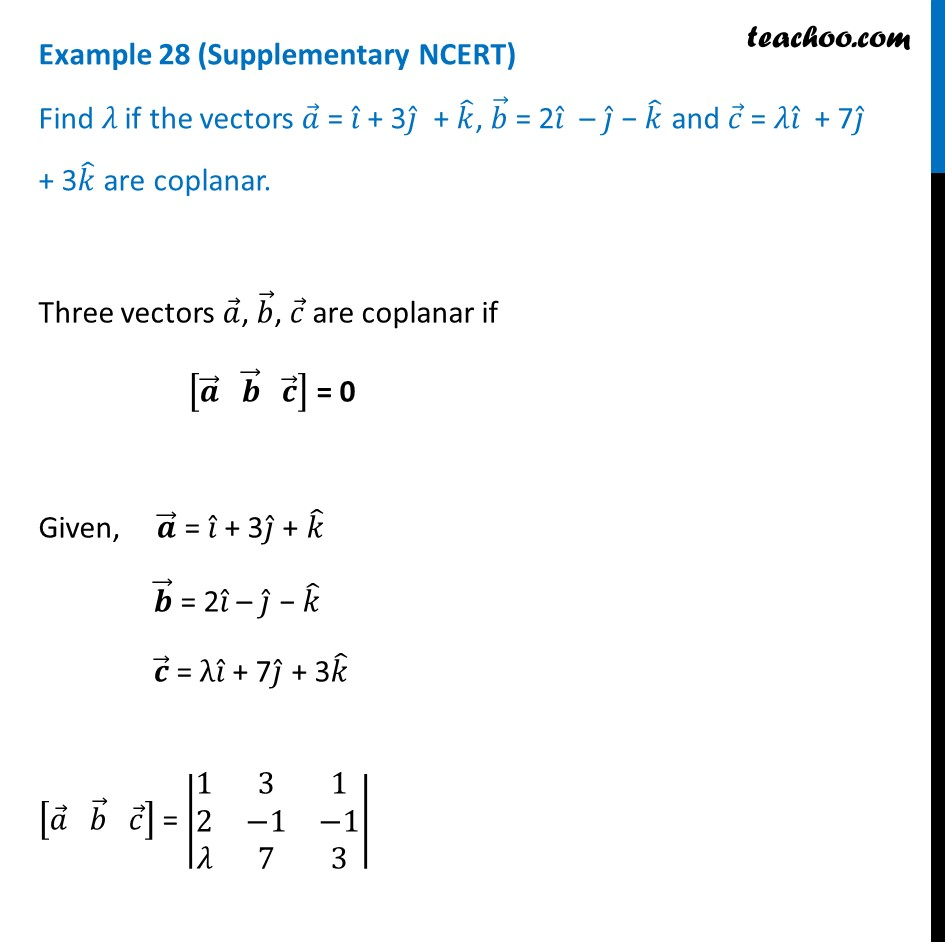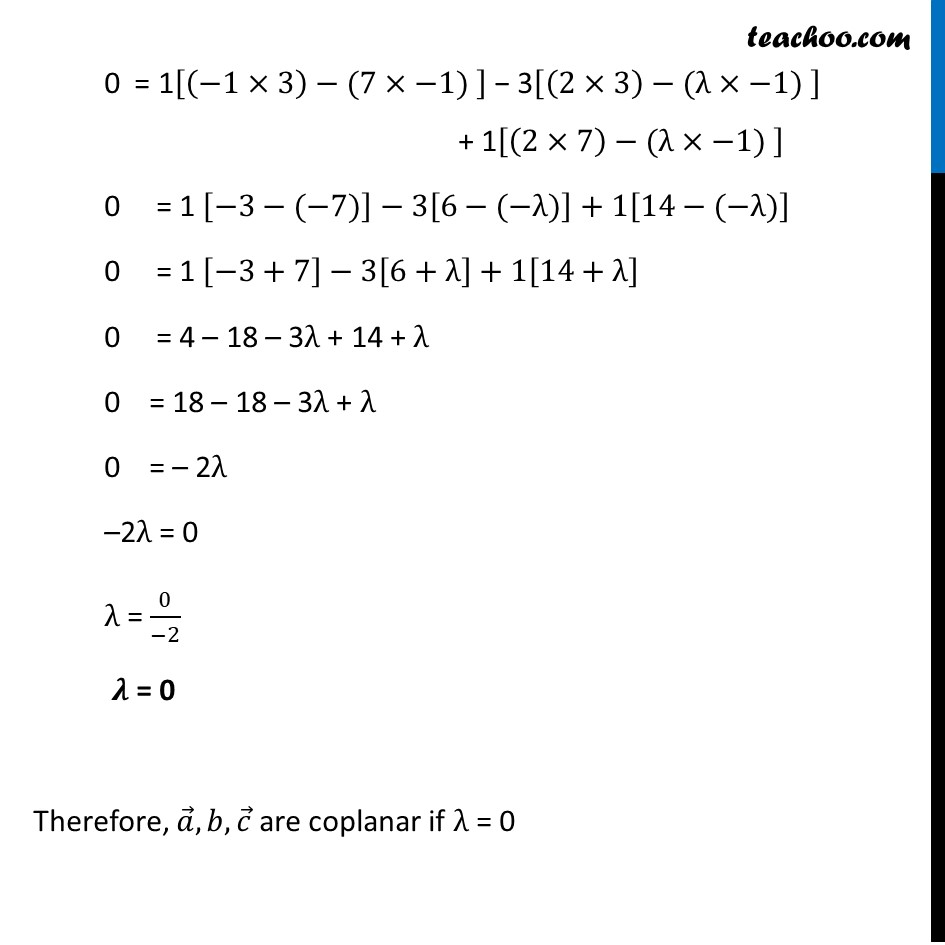Examples

Chapter 10 Class 12 Vector Algebra
Serial order wiseLearn in your speed, with individual attention - Teachoo Maths 1-on-1 Class

### Transcript

Example 28 (Supplementary NCERT) Find 𝜆 if the vectors 𝑎 ⃗ = 𝑖 ̂ + 3𝑗 ̂ + 𝑘 ̂, 𝑏 ⃗ = 2𝑖 ̂ – 𝑗 ̂ − 𝑘 ̂ and 𝑐 ⃗ = 𝜆𝑖 ̂ + 7𝑗 ̂ + 3𝑘 ̂ are coplanar.Three vectors 𝑎 ⃗, 𝑏 ⃗, 𝑐 ⃗ are coplanar if [𝒂 ⃗" " 𝒃 ⃗" " 𝒄 ⃗ ] = 0 Given, 𝒂 ⃗ = 𝑖 ̂ + 3𝑗 ̂ + 𝑘 ̂ 𝒃 ⃗ = 2𝑖 ̂ – 𝑗 ̂ − 𝑘 ̂ 𝒄 ⃗ = λ𝑖 ̂ + 7𝑗 ̂ + 3𝑘 ̂ [𝑎 ⃗" " 𝑏 ⃗" " 𝑐 ⃗ ] = |■8(1&3&[email protected]&−1&−1@𝜆&7&3)| 0 = 1[(−1×3)−(7×−1) ] − 3[(2×3)−(λ×−1) ] + 1[(2×7)−(λ×−1) ] 0 = 1 [−3−(−7)]−3[6−(−λ)]+1[14−(−λ)] 0 = 1 [−3+7]−3[6+λ]+1[14+λ] 0 = 4 – 18 – 3λ + 14 + λ 0 = 18 – 18 – 3λ + λ 0 = – 2λ –2λ = 0 λ = 0/(−2) 𝝀 = 0 Therefore, 𝑎 ⃗,𝑏,𝑐 ⃗ are coplanar if λ = 0